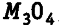# Two oxides of a metal contain 27.6% and 30.0% of oxygen respectively

Two oxides of a metal contain 27.6% and 30.0% of oxygen respectively. If the formula of the first oxide is, find that of the second.

In the first oxide, oxygen = 27.6
Metal 100— 27.6= 72.4 parts by mass.
As the formula of the oxide is \$M_{3}O_{4}\$, it means 72.4 parts by mass of metal = 3 atoms of metal and 4 atoms of oxygen = 27.6 parts by mass.
In the second oxide, oxygen = 30.0 parts by mass and metal = 100 - 30 = 70 parts by mass.
But 72.4 parts by mass of metal = 3 atoms of metal.
70 parts by mass of metal = 3 / 72.4 x 70 atoms of metal = 2.90 metals of atoms.
Also, 27.6 part by mass of oxygen = 4 atoms of oxygen
30 part by mass of oxygen = 4/27.6 x 30 atoms of metal = 4.35 metals of atoms.
Hence, ratio of M : O in the second oxide
= 2.90:4.35 = 1 : 1.5 or 2:3
Formula of the other metal oxide is \$M_{2}O_{3}\$.